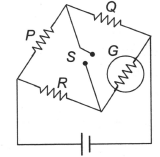The figure shows a circuit diagram of a Wheatstone bridge to measure the resistance G of the galvanometer. The relation PQ=RG  will be satisfied only when

# The figure shows a circuit diagram of a Wheatstone bridge to measure the resistance G of the galvanometer. The relation $\frac{\mathrm{P}}{\mathrm{Q}}=\frac{\mathrm{R}}{\mathrm{G}}$  will be satisfied only when1. A

the galvanometer shows a deflection when switch S is closed

2. B

the galvanometer shows a deflection when switch S is open

3. C

the galvanometer shows no change in deflection whether S is open or closed

4. D

the galvanometer shows no deflection

Register to Get Free Mock Test and Study Material

+91

Verify OTP Code (required)

### Solution:

In balance condition, no current will flow through the branch containing S.Register to Get Free Mock Test and Study Material

+91

Verify OTP Code (required)